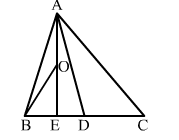# In ∆ABC, it is given that D is the midpoint of BC; E is the midpoint of BD and O is the midpoint of AE.

Question:

In ∆ABC, it is given that D is the midpoint of BCE is the midpoint of BD and O is the midpoint of AE. Then, ar(∆BOE) = ?

(a) $\frac{1}{3} \operatorname{ar}(\Delta A B C)$

(b) $\frac{1}{4} \operatorname{ar}(\Delta A B C)$

(c) $\frac{1}{6} \operatorname{ar}(\Delta A B C)$

(d) $\frac{1}{8} \operatorname{ar}(\Delta A B C)$Solution:

(d) $\frac{1}{8} \operatorname{ar}(\Delta A B C)$

Given: D is the midpoint of BCE is the midpoint of BD and O is the mid point of AE.
Since D is the midpoint of BC, AD is the median of ∆ABC.
E is the midpoint of BC, so AE is the median of ∆ABDO is the midpoint of AE, so BO is median of ABE.
We know that a median of a triangle divides it into two triangles of equal areas.

i.e., $\operatorname{ar}(\Delta A B D)=\frac{1}{2} \times \operatorname{ar}(\Delta A B C)$            ...(i)

$\operatorname{ar}(\Delta A B E)=\frac{1}{2} \times \operatorname{ar}(\Delta A B D)$                   ...(ii)

$\operatorname{ar}(\Delta B O E)=\frac{1}{2} \times \operatorname{ar}(\Delta A B E)$                  ...(iii)

From (i), (ii) and (iii), we have:

$\operatorname{ar}(\Delta B O E)=\frac{1}{2} \operatorname{ar}(\Delta A B E)$

$\operatorname{ar}(\Delta B O E)=\frac{1}{2} \times \frac{1}{2} \times \frac{1}{2} \times \operatorname{ar}(\Delta A B C)$

$\therefore \operatorname{ar}(\Delta B O E)=\frac{1}{8} \operatorname{ar}(\Delta A B C)$